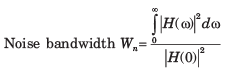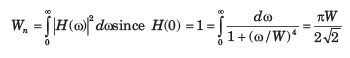Courses

# Random Process - MCQ Test

## 20 Questions MCQ Test GATE ECE (Electronics) 2022 Mock Test Series | Random Process - MCQ Test

Description
This mock test of Random Process - MCQ Test for Electronics and Communication Engineering (ECE) helps you for every Electronics and Communication Engineering (ECE) entrance exam. This contains 20 Multiple Choice Questions for Electronics and Communication Engineering (ECE) Random Process - MCQ Test (mcq) to study with solutions a complete question bank. The solved questions answers in this Random Process - MCQ Test quiz give you a good mix of easy questions and tough questions. Electronics and Communication Engineering (ECE) students definitely take this Random Process - MCQ Test exercise for a better result in the exam. You can find other Random Process - MCQ Test extra questions, long questions & short questions for Electronics and Communication Engineering (ECE) on EduRev as well by searching above.
QUESTION: 1

### Consider a low-pass random process with a white-noise power spectral density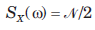as shown in fig. ​ ​ ​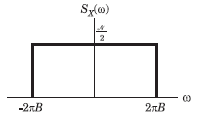Que: The auto correlation function Rx(τ) is

Solution: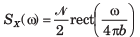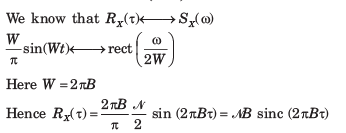QUESTION: 2

### Consider a low-pass random process with a white-noise power spectral densityas shown in fig.Que: The power PX is

Solution: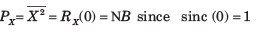QUESTION: 3

### If X(t) is a stationary process having a mean value E[X(t)] = 3 and autocorrelation function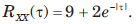The variance of random variable Y =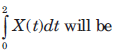Solution: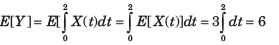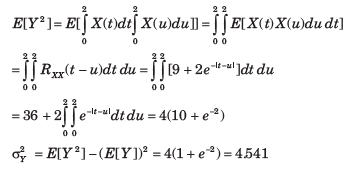QUESTION: 4

A random process is defined by X(t) = Acos(πt) where A is a gaussian random variable with zero mean and variance σπ2. The density function of X(0)

Solution: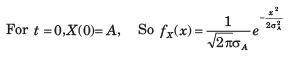QUESTION: 5

The two-level semi-random binary process is defined by X(t) = A or -A

where (n-1)T < t < nt and the levels A and -A occur with equal probability. T is a positive constant and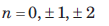Que: The mean value E[X(t)] is

Solution: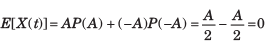QUESTION: 6

The two-level semi-random binary process is defined by X(t) = A or -A

where (n-1)T < t < nt and the levels A and -A occur with equal probability. T is a positive constant andQue: The auto correlation Rxx = (t1 = 0.5T, t2 = 0.7 T) will be

Solution: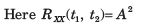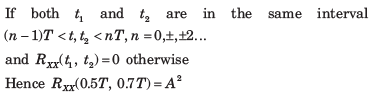QUESTION: 7

A random process consists of three samples function X(t, s1 ) = 2, X(t, s2 ) = 2cos t1  and X(t, s3 ) = 3sint  - each occurring with equal probability. The process is

Solution: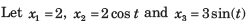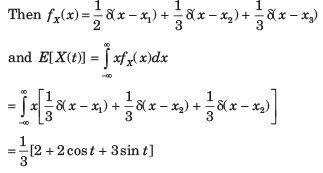The mean value is time dependent so X (t) is not stationary in any sense.

QUESTION: 8

The auto correlation function of a stationary ergodic random process is shown in fig.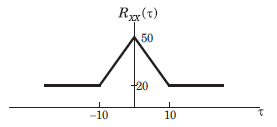Que: The mean value E[X(t)] is

Solution:

We know that for ergodic with no periodic component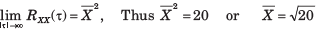QUESTION: 9

The auto correlation function of a stationary ergodic random process is shown in fig.Que: The E[X2(t)] is

Solution: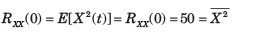QUESTION: 10

The auto correlation function of a stationary ergodic random process is shown in fig.Que: The variance σx is

Solution: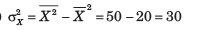QUESTION: 11

A stationary zero mean random process X(t) is ergodic has average power of 24 W and has no periodic component. The valid auto correlation function is

Solution:

For (A) : It has a periodic component.
For (B) ; It is not even in τ, total power is also incorrect.
For (C) It depends on t not even in τ and average power is ∞

QUESTION: 12

A stationary random process X(t) is applied to the input of a system for which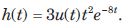If E[X(t)] = 2, the mean value of the system's response Y(t) is

Solution: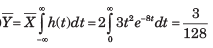QUESTION: 13

A random process X(t) is applied to a network with impulse response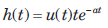where a > 0 is a constant. The cross correlation of X(t) with the output Y(t) is known to have the same form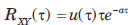Que: The auto correlation of Y(t) is

Solution: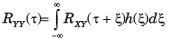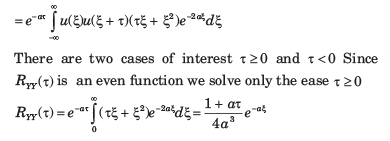QUESTION: 14

A random process X(t) is applied to a network with impulse responsewhere a > 0 is a constant. The cross correlation of X(t) with the output Y(t) is known to have the same formQue: The average power in Y(t) is

Solution: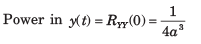QUESTION: 15

A random noise X(t) having a power spectrum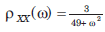is applied to a differentiator that has a transfer function H(ω) =  j(ω). The output is applied to a network for which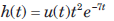Que : The average power in X(t) is

Solution: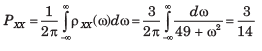QUESTION: 16

A random noise X(t) having a power spectrumis applied to a differentiator that has a transfer function H(ω) =  j(ω). The output is applied to a network for whichQue : The power spectrum of Y(t) is

Solution: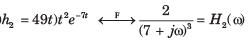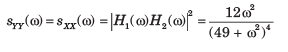QUESTION: 17

White noise with power density N0 /2 is applied to a low pass network for which |H(0)| = 2. It has a noise bandwidth of 2 MHz. If the average output noise power is 0.1 W in a 1 - Ω( resistor, the value of Nis

Solution: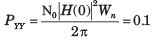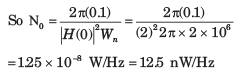QUESTION: 18

An ideal filter with a mid-band power gain of 8 and bandwidth of 4 rad/s has noise X(t) at its input with power spectrum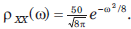The noise power at the network's output is (F(2) = 0.9773)

Solution: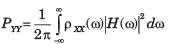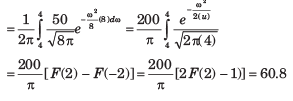QUESTION: 19

White noise with power density N0 /2 = 6 μW/Hz is applied to an ideal filter of gain 1 and bandwidth W rad/s. If the output's average noise power is 15 watts, the bandwidth W is

Solution: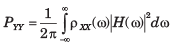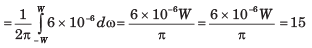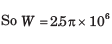QUESTION: 20

A system have the transfer function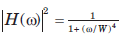where W is a real positive constant. The noise bandwidth of the system is

Solution: